# Side Window Filtering 论文解读和C++实现

2019/08/23 09:37

Side Window Filtering

https://github.com/Ldpe2G/ArmNeonOptimization/tree/master/sideWindowBoxFilter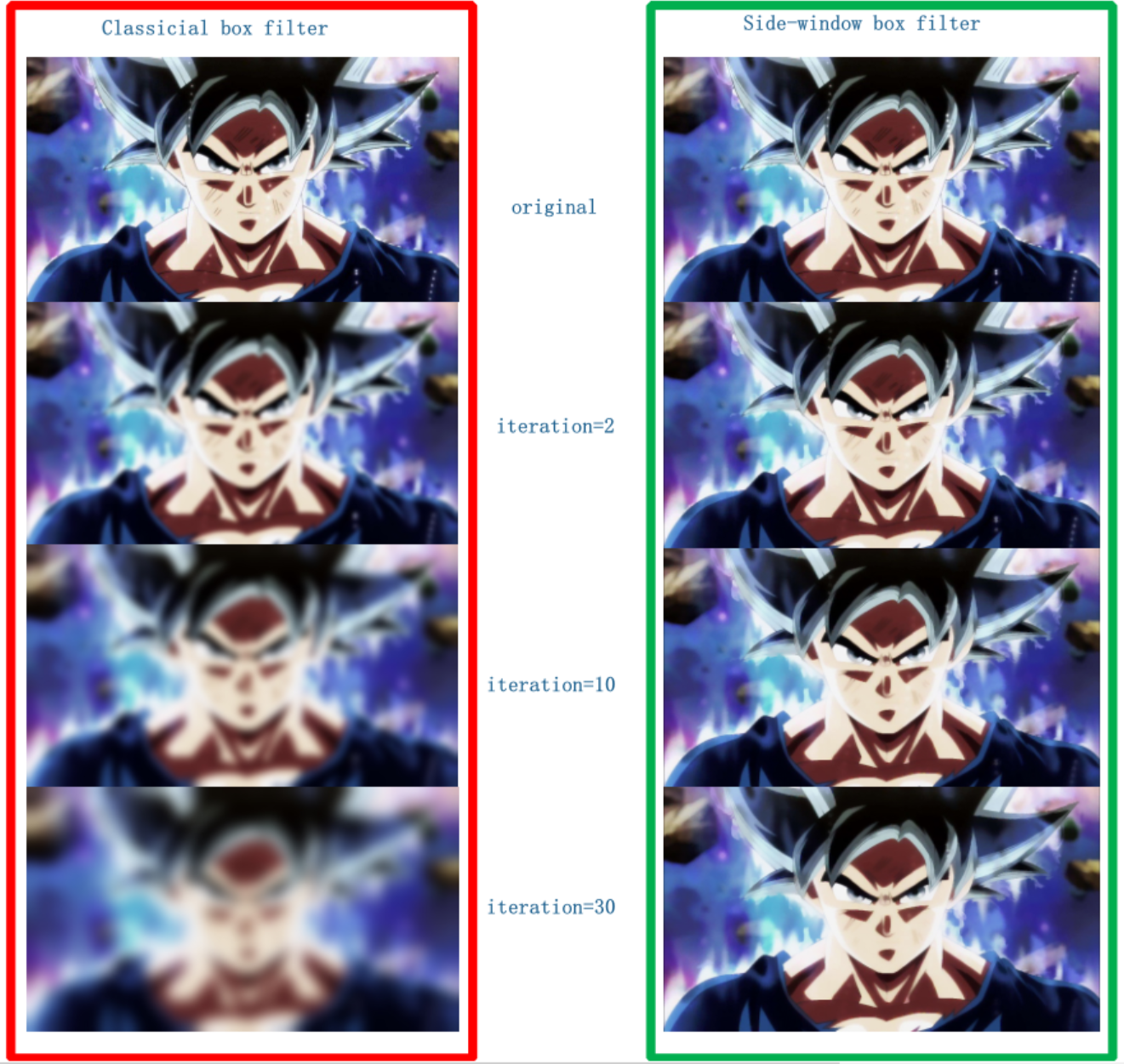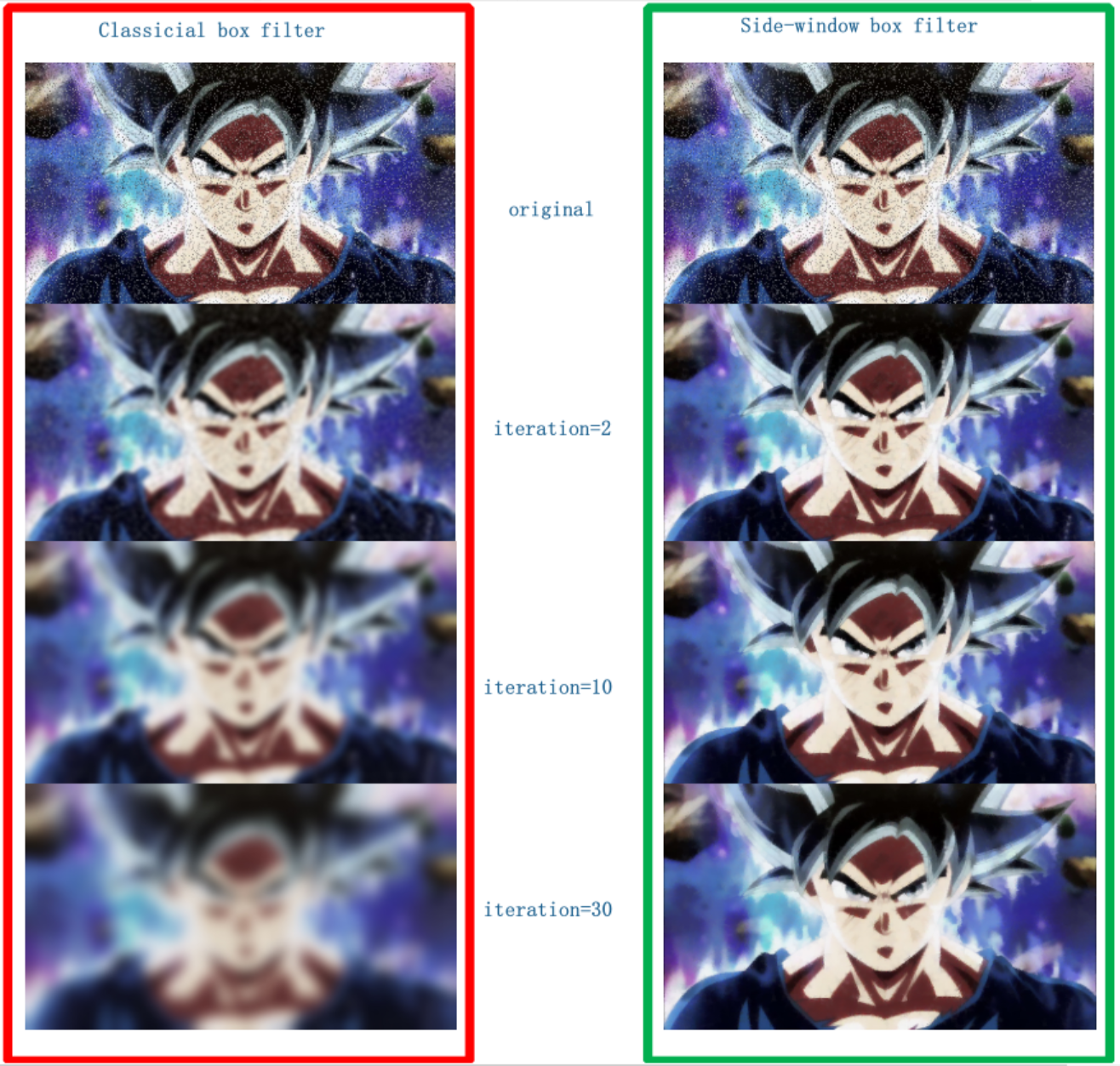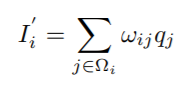Ω是以像素点 i 为中心的滤波窗口，w是滤波权值，q是原图像素值，I'是输出结果。

（这里的边缘不是指图片的大小边界，而是指图像中物体的边缘）的话，以像素为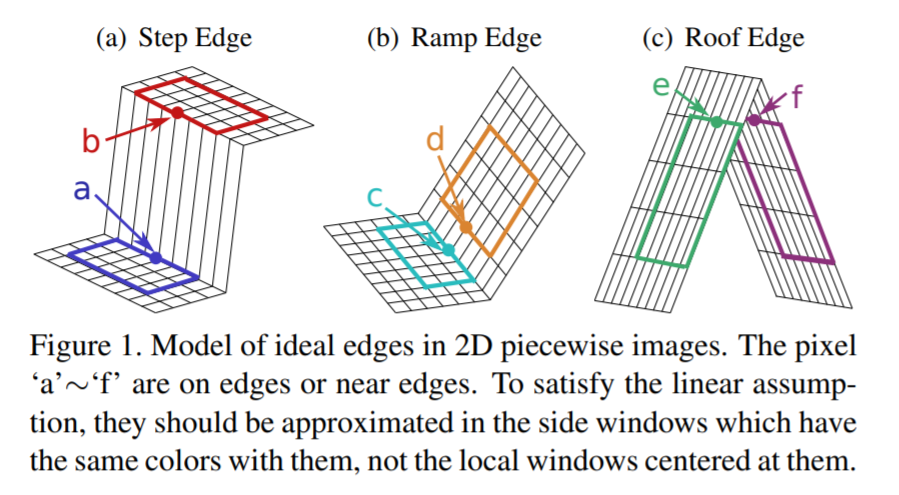(a)阶梯状边缘、(b)斜坡状边缘和(c)屋顶状边缘。论文中也给出了这3三种边缘的形象展示：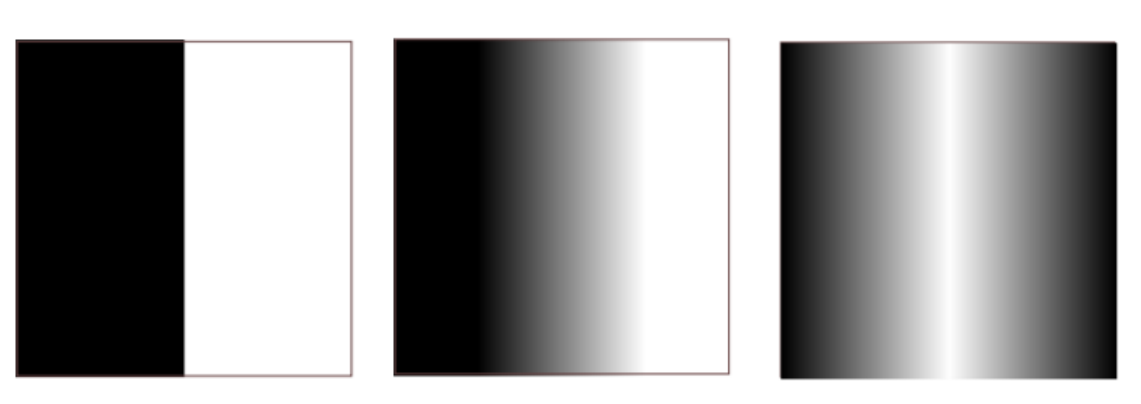g'(x - ε, y) ≠ g'(x + ε, y)，导数也不等由于边缘部分的跳跃。因此对于这两块区域的泰勒展开也是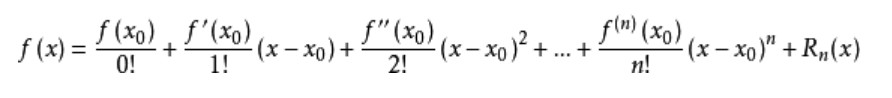“泰勒公式是将一个在 x=x0 处具有n阶导数的函数 f(x) 利用关于 (x - x0) 的n次多项式来

g(x - 2ε, y) ≈ f(x0) + f'(x0)(x - x0)

= g(x - ε, y) + g'(x - ε, y)(x - 2ε - (x - ε))

= g(x - ε, y) + g'(x - ε, y)(- ε)

g(x + 2ε, y) ≈ f(x0) + f'(x0)(x - x0)

= g(x + ε, y) + g'(x + ε, y)(x + 2ε - (x + ε))

= g(x + ε, y) + g'(x + ε, y)ε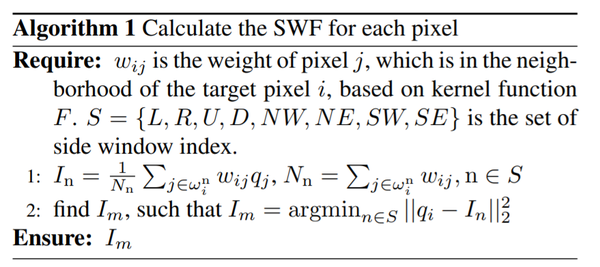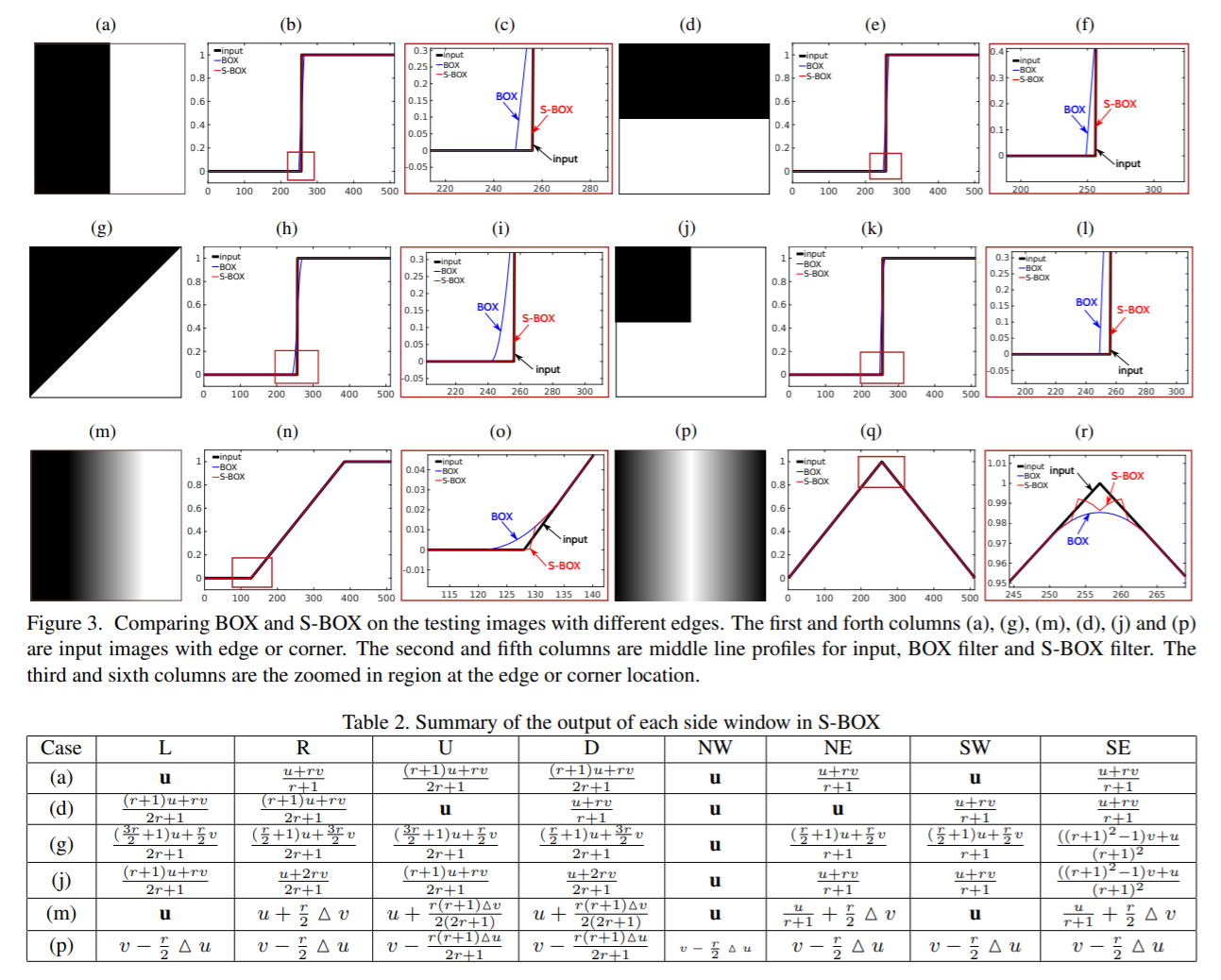CVPR2019 Oral论文 #5176 Side Window Filtering介绍

Sub-window Box Filter论文介绍

0
0 收藏

### 作者的其它热门文章not an existing directory.

-- Configuring incomplete, errors occurred!
2020/05/04 22:32

git clone --recursive xxx
2020/06/24 21:57

2 评论
0 收藏
0